# populR: a Package for Population Downscaling in R

Population data provision is usually framed by regulations and restrictions and hence spatially aggregated in predefined enumeration units such as city blocks and census tracts. Many applications require population data at finer scale, and therefore, one may use downscaling methods to transform population counts from coarse spatial units into smaller ones. Although numerous methods for downscaling of population data have been reported in the scientific literature, only a limited number of implementation tools exist. In this study, we introduce populR, an R package that responds to this need. populR provides two downscaling methods, namely Areal Weighted Interpolation and Volume Weighted Interpolation, which are illustrated and compared to alternative implementations in the sf and areal packages using a case study from Mytilini, Greece. The results provide evidence that the vwi approach outperforms the others, and thus, we believe R users may gain significant advantage by using populR for population downscaling.

Marios Batsaris https://www.mbatsaris.gr (University of the Aegean) , Dimitris Kavroudakis https://www.britannica.com/animal/bilby (University of the Aegean)
2023-02-10

# 1 Introduction

Information about the spatial distribution of the population is crucial in addressing a wide range of geographical information science problems . One of the most common sources of population data is the Census of Population . In the EU, censuses are carried out every ten years and are regulated by the EU 763/2008 law . During the census, detailed information about the population is collected with a high level of granularity. This information is confidential, and due to personal data laws and privacy concerns, census data are de-identified via spatial aggregation into coarse administrative units.

Aggregated representations of census data may not reflect the spatial heterogeneity of the population within the reported administrative units, and therefore, one may need to use downscaling methods to transform population counts from the predefined coarse boundaries (source) into finer-scale sub-units (target). Areal interpolation is broadly used in population downscaling .

Areal interpolation methods can be categorized according to usage of additional information such as land use data and night lights to guide the interpolation process. Ancillary information independent methods are further distinguished in: a) point-based and b) area-based . Areal Weighted Interpolation (AWI) is one of the most common methods of areal interpolation of population without exploiting ancillary information [Comber and Zeng (2019); Kim and Yao (2010);] in a Geographic Information System (GIS). AWI guides the interpolation process on the basis of areal weights calculated by the area of intersection between the source and target features . This method has four main advantages: a) It is easy and straightforward to implement; b) it does not require ancillary information; c) it preserves the initial volume (i.e., the total population within the source zone); and d) it may be implemented in both vector and raster environments . On the other hand, one of the main disadvantages of AWI is its assumption of homogeneity within source zone features .

sf and areal provide AWI functionality in R. sf comes up with a formula either for extensive (population) or intensive (population density) interpolation that calculates the areal weights based on the total area of the source features (total weights), which makes it suitable for completely overlapping data. areal extends sf functionality by providing an additional formula for weight calculation for data without complete overlap. In this case, areal weights are calculated using the sum of the remaining source areas after the intersection (sum weights) .

When the case involves downscaling of urban population data (small scale applications) where the source features (such as city blocks or census tracts) are somehow larger than target features (such as buildings) in terms of footprint area, the sf functionality is unable to calculate the areal weights correctly. Additionally, areal may be confusing for novice R (or GIS) users as it is not obvious that the weight option should be set to sum to calculate areal weights properly.

To overcome such limitations, we introduce populR , a package for downscaling of population data using areal interpolation methods. populR provides an AWI approach that matches areal functionality using sum weights and a VWI (Volume Weighted Interpolation) approach that uses the area of intersection between source and target features multiplied by the building height or number of floors (volume) to guide the interpolation process.

The remainder of the paper is structured as follows. The next section lists the methods included in the populR package, further explains and demonstrates using examples. The numerical experiments section illustrates and discusses the results obtained by populR and compares the results to other alternatives in R. Finally, the paper concludes with a summary, along with future improvements, in the conclusions section.

# 2 The populR package

populR is available to R users through the CRAN and Github repositories and may be installed as shown in the code block below.

# CRAN installation
install.packages("populR")

# Github installation
devtools::install_github("mbatsaris/populR")

The populR functionality focuses on three main pillars, namely downscaling, rounding, and comparing. Additionally, sample data (objects of class sf ) representing city blocks, including population counts (src) and individual buildings attributed by the number of floors (trg) from a small part of the city of Mytilini, Greece, are also available for further experimentation with the package’s functionality.

## Downscaling

Population downscaling is carried out by the primary pp_estimate function.

Suppose a set of $$j = 1, ..., J$$ city block polygons (source) accompanied by population counts and an incongruent yet superimposed set of $$i = 1, ..., B_j$$ individual building polygons (target), along with their characteristics such as footprint area and/or number of floors (or height), the pp_estimate, aims to transform population counts from the source to the target using AWI and VWI approaches. The mathematics behind both approaches is presented in the next two subsections and graphically illustrated in figure 1.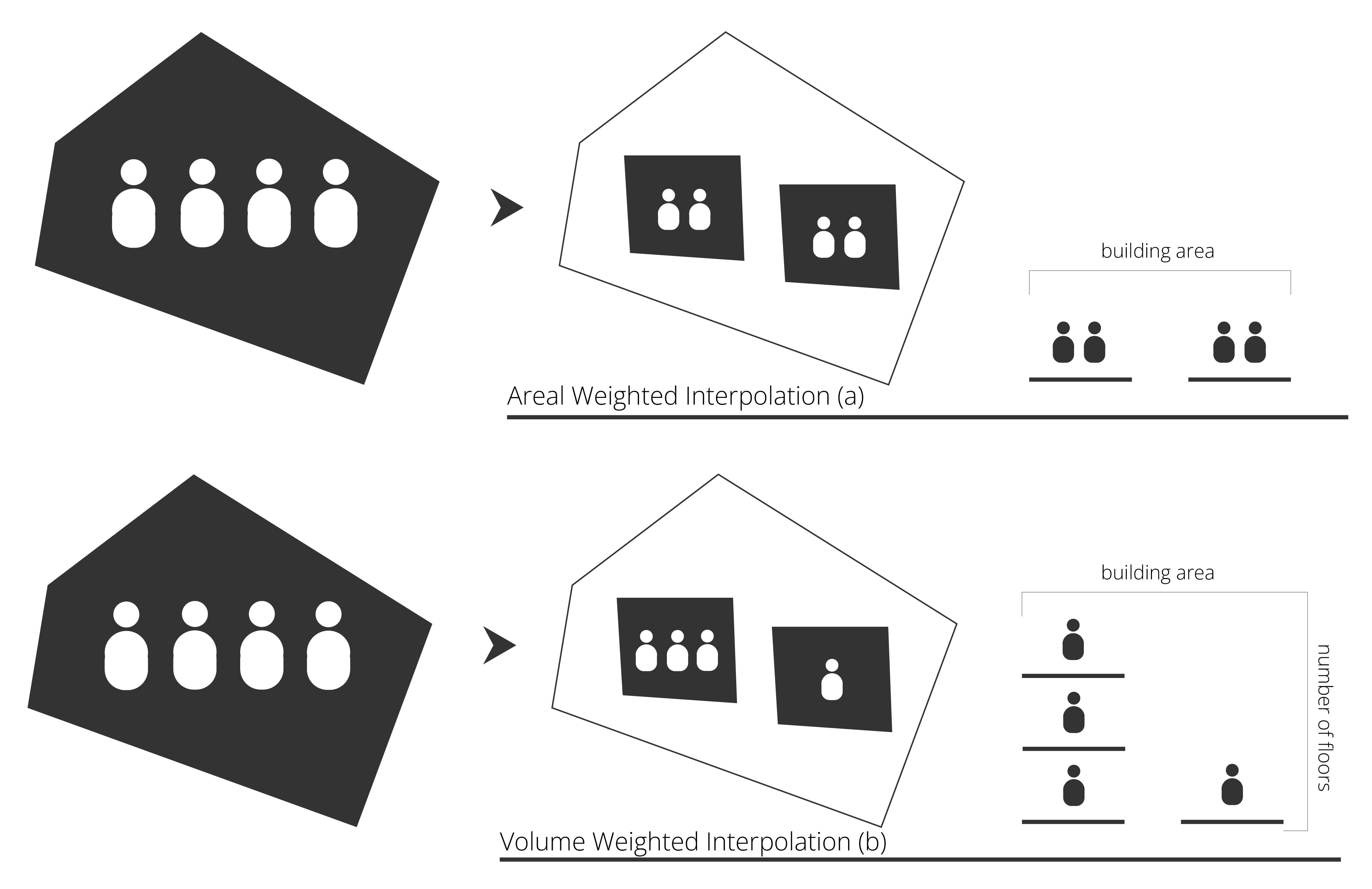Figure 1: Illustration of the populR package’s functionality. populR uses two areal interpolation methods to convert population values from city block polygons (left) to a set of individual building polygons (center). AWI (top) solely relies on the area of intersection between blocks and buildings (top-right). VWI (bottom) uses the area of intersection between blocks and buildings multiplied by the height or number of floors to aid the transformation process (bottom-right).

### AWI

AWI proportionately interpolates the population value of the source features based on areal weights calculated by the area of intersection between the source and target zones and algebraically explained by equations (1) and (2) . In equation (1), areal weights $$w^a_{ij}$$ are calculated by standardizing the measured footprint area of individual building $$i$$ in block $$j$$ ($$a_{ij}$$) over the sum of building footprint areas in the $$j-th$$ city block. Finally, building population values for building $$i$$ in block $$j$$ ($$p_{ij}$$) may be calculated by multiplying the areal weights of building $$i$$ in block $$j$$ with the population value of $$j-th$$ block ($$P_j$$).

$\begin{equation} w_{ij}^a = \frac{a_{ij}}{\sum_{i=1}^{B_{j}} a_{ij}} \text{ , } \tag{1} \end{equation}$

$\begin{equation} p_{ij}^a = w_{ij}^a \times P_j \text{ . } \tag{2} \end{equation}$

A demonstration of the AWI approach is presented in the code block below. In the first line of code, populR is attached to the script. The next two lines load the package’s built-in data, and the last line demonstrates the usage of the pp_estimate function. As a result, pp_estimate returns an object of class sf including individual buildings (target) with population estimates.

# attach package
library(populR)

data('src')
data('trg')

# downscaling using awi
awi <- pp_estimate(target = trg, source = src, spop = pop, sid = sid,
method = awi)

Where:

1. target: An object of class sf that is used to interpolate data to. Usually, target may include polygon features representing building units
2. source: An object of class sf including data to be interpolated. Source may be a set of coarse polygon features, such as city blocks or census tracts
3. sid: Source identification number
4. spop: Source population values to be interpolated
5. method: Two methods provided: AWI (Areal Weighted Interpolation) and VWI (Volume Weighted Interpolation). AWI proportionately interpolates the population values based on areal weights calculated by the area of intersection between the source and target zones. VWI proportionately interpolates the population values based on volume weights calculated by the area of inter- section between the source and target zones multiplied by the volume information (height or number of floors)

### VWI

Given the number of floors (or height) of individual buildings, VWI applies the same logic as AWI. Instead of areal weights, VWI proportionately dis-aggregates source population counts using volume weights measured by the area of intersection multiplied either by individual building height (if available) or number of floors. VWI may be mathematically expressed by equations (3) to (5). First, the volume of building $$i$$ in block $$j$$ ($$v_{ij}$$) is measured by multiplying the footprint area ($$a_{ij}$$) of building $$i$$ in block $$j$$ with the number of floors or height ($$n_{ij}$$) as shown in equation (3). Next, volume weights ($$w^v_{ij}$$) are calculated by standardizing the volume of building $$i$$ in block $$j$$ ($$v_{ij}$$) by the sum of the total volume of buildings in the $$j-th$$ block (4). Then, building population values for building $$i$$ in block $$j$$ ($$p^v_{ij}$$) may be calculated by multiplying the volume weights ($$w^v_{ij}$$) of building $$i$$ in block $$j$$ with the population value of block $$j$$ ($$P_J$$) as shown in (5).

$\begin{equation} v_{ij} = a_{ij} \times n_{ij} \text{ , } \tag{3} \end{equation}$

$\begin{equation} w_{ij}^v = \frac{v_{ij}}{\sum_{i=1}^{B_{j}} v_{ij}} \text{ , } \tag{4} \end{equation}$

$\begin{equation} p_{ij}^v = w_{ij}^v \times P_j \text{ . } \tag{5} \end{equation}$

The code block below provides an example of the VWI approach.

# downscaling using vwi
vwi <- pp_estimate(target = trg, source = src, spop = pop, sid = sid,
volume = floors, method = vwi)

Where:

1. volume: Target feature volume information (height or number of floors). Required when method is set to vwi

It is important to mention that when volume information (number of floors or building height) is not available for the entire target dataset, users may replace missing values with $$1$$ if they want to include them as buildings with 1 floor; otherwise, users may replace missing values with $$0$$ if they want to exclude them from the downscaling process.

## Rounding

AWI is volume-preserving (as VWI) and returns an object of class sf with decimal population counts for each target feature. To increase the readability of the results as well as to maintain the initial source population values, it is essential for many applications to provide integer values by rounding off to the closest integer. This transformation may result in a shortage or surplus in comparison to the initial values (source values), and therefore, to cope with this problem, the pp_round function is proposed.

First, estimated population values are converted into integer numbers. Next, the pp_round function calculates the differences between the initial population counts and the sum of the integer values for each city block; it is activated only if the quantified difference is either positive or negative. In either case, during this process, target-based differences are also calculated and used to refine the integer counts by adding or removing one population unit in order to preserve the initial source counts when summed up.

An example of the pp_round function is provided below.

# downscaling using awi
awi <- pp_round(x = awi, tpop = pp_est, spop = pop, sid = sid)

# downscaling using awi
vwi <- pp_round(x = vwi, tpop = pp_est, spop = pop, sid = sid)

Where:

1. x: An object of class sf obtained by the pp_estimate function
2. tpop: Target population estimates obtained by the pp_estimate function
3. spop: Initial source population values (included after the implementation of the pp_estimate function)
4. sid: Source identification number

As a result, pp_round returns an object of class sf with integer estimates of population values with respect to the initial source population values.

## Comparing

Volume-preserving means that estimated values should sum up to the initial population counts for each source unit, and therefore, one may need to compare target estimates to the initial source values. For this purpose, a function is introduced under the alias pp_compare. The pp_compare function measures the root mean squared error (RMSE), the mean absolute error (MAE), and finally, the statistical relationship between the initial source values and the estimated ones, which is calculated and depicted on a 2D scatter diagram along with the value of the correlation coefficient $$R^2$$.

A short example of the pp_compare function is presented in the code block below, where src_awi (and src_vwi) is a data.frame created by grouping the sum of the estimated values along with the initial population values for each source feature.

# attach library
library(dplyr)

# group estimated and actual values by source identification number - awi
src_awi <- awi %>%
group_by(sid) %>%
summarise(est = sum(pp_est), act = unique(pop))

# awi approach compare to source values
pp_compare(x = src_awi, estimated = est, actual = act, title = "awi vs source")

# group estimated and actual values by source identification number - vwi
src_vwi <- vwi %>%
group_by(sid) %>%
summarise(est = sum(pp_est), act = unique(pop))

# vwi approach compare to source values
pp_compare(x = src_vwi, estimated = est, actual = act, title = "vwi vs source")

Where:

1. x: An object of class sf or a data.frame including estimated and actual values
2. estimated: Target population estimates obtained by the pp_estimate function
3. actual: Actual population values
4. title: Scatterplot title (quoted)

pp_compare returns a list of elements with RMSE, MAE, linear regression, and $$R^2$$ coefficient information.

# 3 Numerical Experimentation

In this section, the results of the populR package are illustrated and further compared to sf and areal. The analysis focus on implementation, comparison to a reference data set, and performance.

## Case study

To examine the efficacy and efficiency of the populR extension based on actual data, a small part of the city of Mytilini was chosen as the subject for a case study (Figure 2). The study area consists of 9 city blocks (src), 179 buildings (trg), and 911 residents.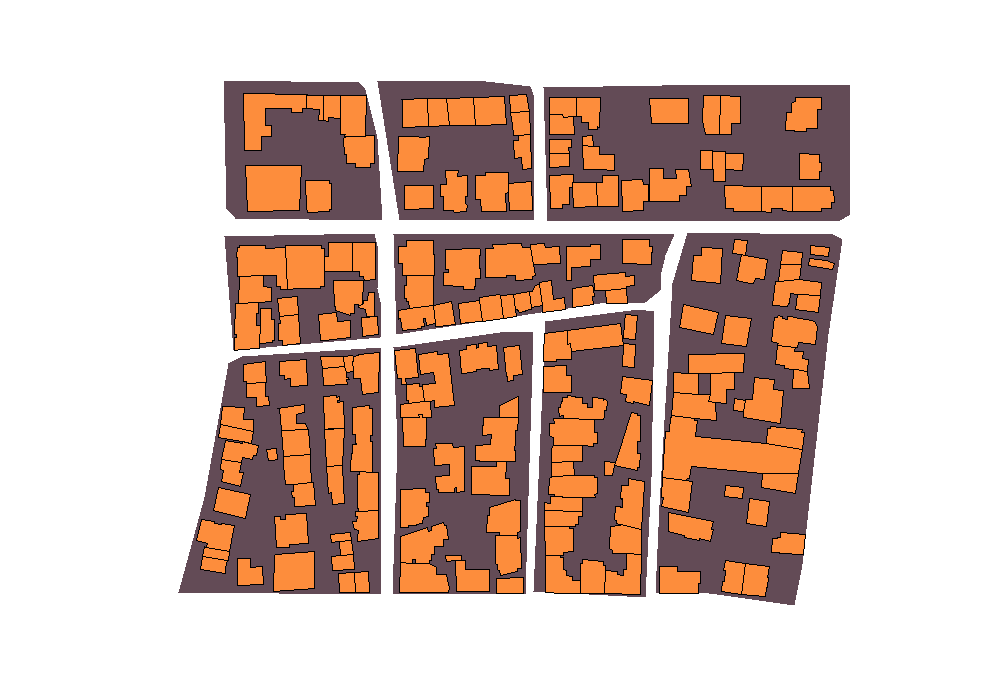Figure 2: Part of the city of Mytilini used as the case study for numerical experiments. The study area is represented by 9 city blocks and 179 buildings (in orange). The study area counts 911 residents.

Table 1: Data used for numerical experiments
Title Format Source Year
City block population counts Tabular Hellenic Statistical Authority 2011
City blocks Spatial Hellenic Statistical Authority 2011
Buildings Spatial Hellenic Statistical Authority 2011

Table 1 presents the data used in this case study. City block population counts were retrieved in tabular format while city blocks (source - src) and buildings (target - trg) were provided in spatial format. City block population counts and city blocks and buildings correspond to the 2011 Census of Housing and Population .

## Implementation

In this section, a demonstration of the sf, areal and populR packages takes place. First, the packages are attached to the script and next, populR built-in data are loaded. Then, areal interpolation implementation follows for each one of the aforementioned packages.

For the reader’s convenience, names were shortened as follows: a) awi: populR AWI approach, b) VWI: populR vwi approach, c) aws: areal using extensive interpolation and sum weights, d) awt: areal using extensive interpolation and total weights, and e) sf: sf using extensive interpolation and total weights.

# attach libraries
library(populR)
library(areal)
library(sf)

data("trg", package = "populR")
data("src", package = "populR")

# populR - awi
awi <- pp_estimate(target = trg, source = src, spop = pop, sid = sid,
method = awi)

# populR - vwi
vwi <- pp_estimate(target = trg, source = src, spop = pop, sid = sid,
volume = floors, method = vwi)

# areal - sum weights
aws <- aw_interpolate(trg, tid = tid, source = src, sid = 'sid',
weight = 'sum', output = 'sf', extensive = 'pop')

# areal - total weights
awt <- aw_interpolate(trg, tid = tid, source = src, sid = 'sid',
weight = 'total', output = 'sf', extensive = 'pop')

# sf - total weights
sf <- st_interpolate_aw(src['pop'], trg, extensive = TRUE)

Evidently, sf requires less arguments than populR and areal, which makes it very easy to implement. populR requires at least 5 arguments, and areal at least 7, which may increase the implementation complexity.

The study area counts 911 residents, as already mentioned in previous section. awi, vwi and aws correctly estimated population values as they sum to 911, while awt and sf underestimated values. This is expected as both methods use the total area of the source features during the interpolation process and are useful when source and target features completely overlap.

Moreover, identical results were obtained by the awi and aws approaches, but somehow different results were obtained by the vwi, as shown in Table 2.

Table 2: Implementation results obtained by awi, vwi, awt, aws and sf for 10 buildings of the study area.
nr awi vwi aws awt sf
1 1.36 0.37 1.36 0.58 0.58
2 1.42 0.39 1.42 0.60 0.60
3 16.12 15.47 16.12 5.99 5.99
4 7.47 4.30 7.47 2.77 2.77
5 2.22 0.61 2.22 0.94 0.94
6 21.08 28.32 21.08 7.83 7.83
7 1.34 0.44 1.34 0.62 0.62
8 2.57 1.45 2.57 1.36 1.36
9 6.54 5.03 6.54 2.75 2.75
10 6.68 3.84 6.68 2.48 2.48

Finally, visual representations of the results are shown in Figure 3.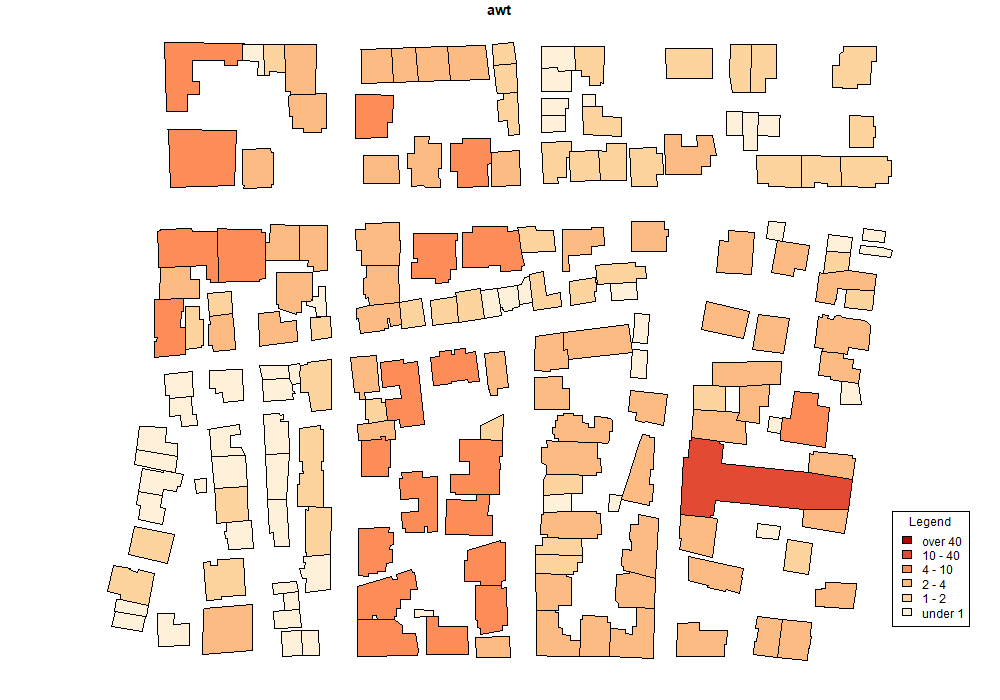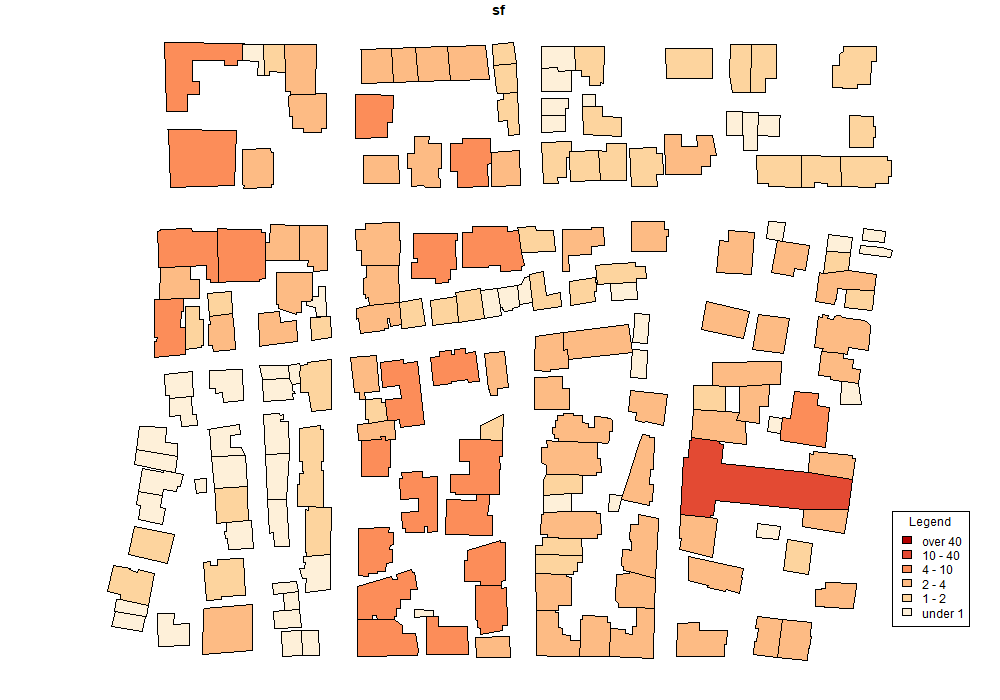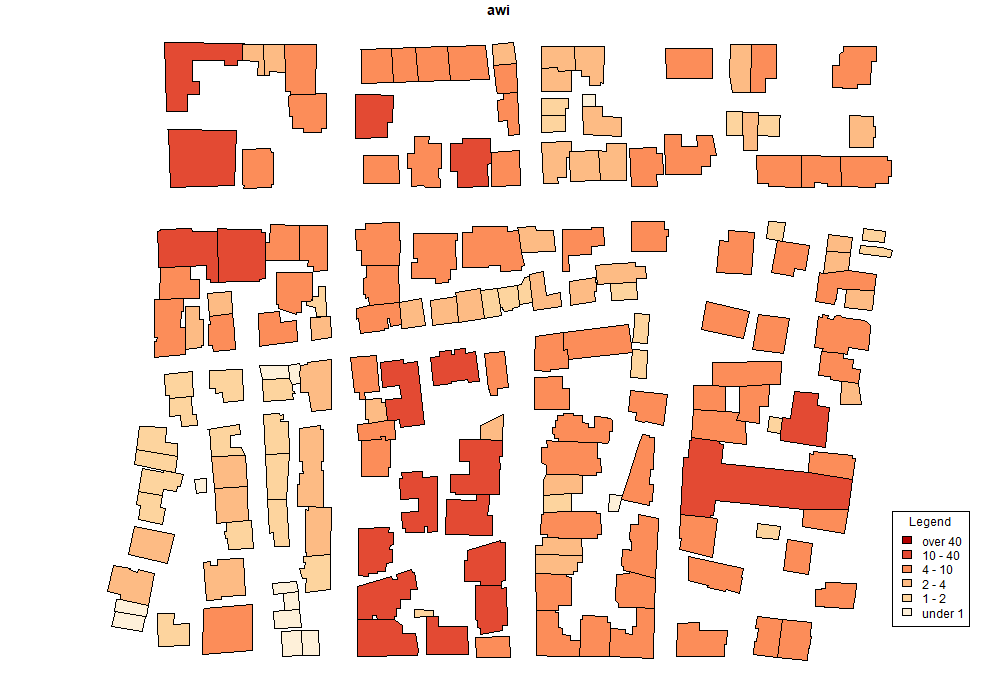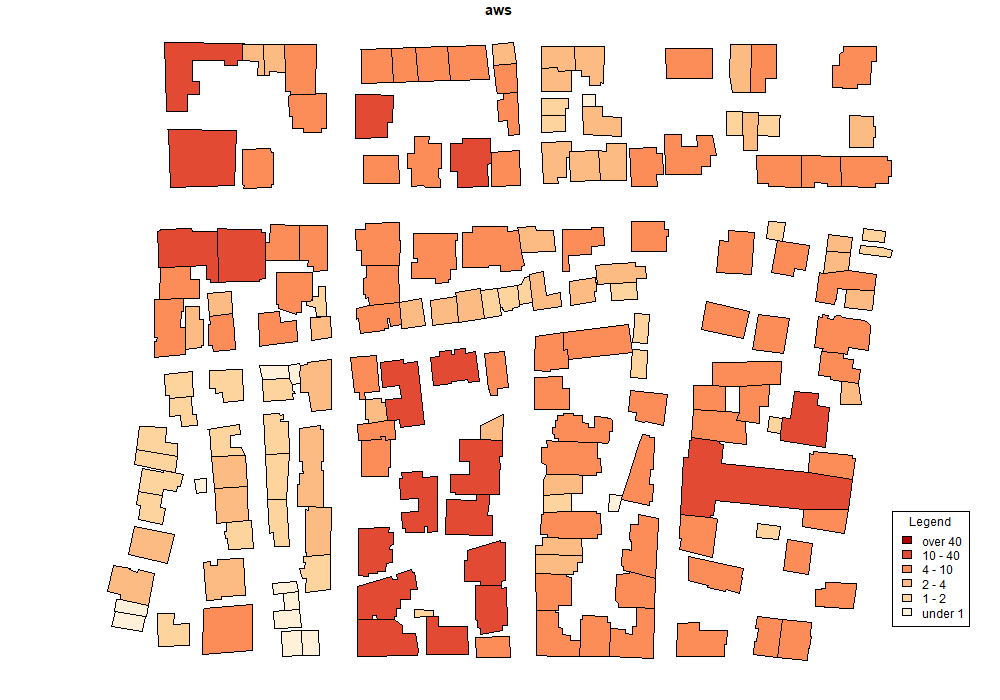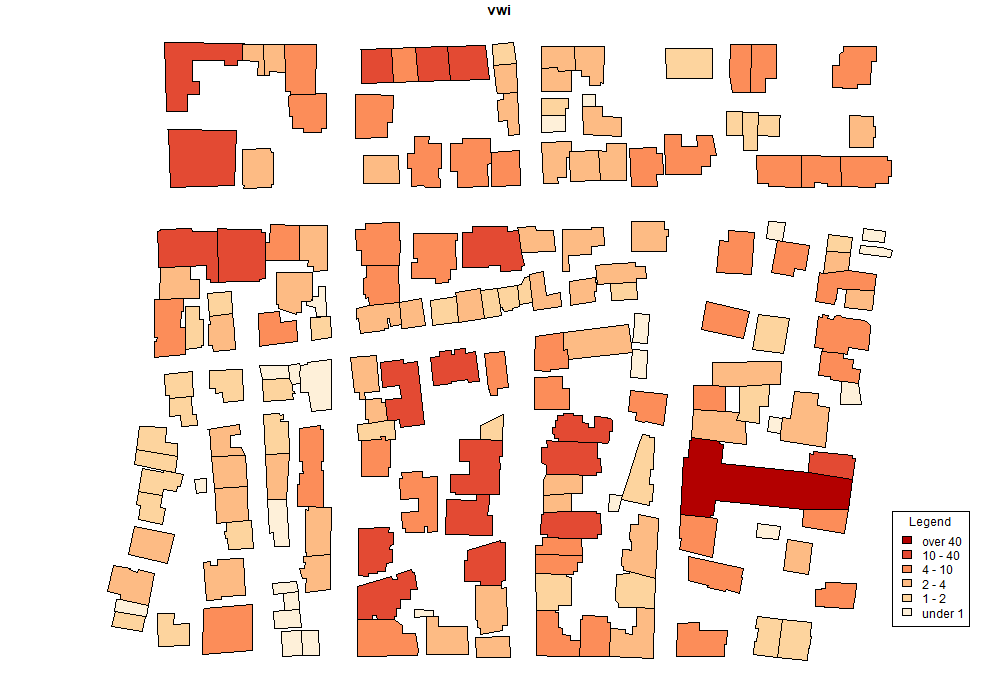Figure 3: Cartographic representation of the downscaling results acquired by a) awt (top-left), b) sf (top-right), c) awi (center-left), d) aws (center-right), and e) vwi (bottom). awt and sf use the same formula therefore, they provide similar results. Additionally, the same results obtained by aws and awi as they are based on the same formula. vwi provide somehow different distribution because of the influence of the volume weights during the interpolation process.

## Comparison to reference data

Due to confidentiality concerns, population data at the granularity of building are not publicly available in Greece. Therefore, an alternate population distribution previously published in Batsaris et al. (2019) was used as reference data (rf). rf population values are also included in the build-in trg data set.

Using the pp_compare function as shown in the example below, we investigated the statistical relationship between rf and the results obtained by populR, areal, and sf packages.

# compare awi to rf
pp_compare(data, estimated = awi, actual = rf, title = "awi vs rf")

# compare vwi to rf
pp_compare(data, estimated = vwi, actual = rf, title = "vwi vs rf")

# compare sf to rf
pp_compare(data, estimated = sf, actual = rf, title = "sf vs rf")

# compare awt to rf
pp_compare(data, estimated = awt, actual = rf, title = "awt vs rf")

# compare aws to rf
pp_compare(data, estimated = aws, actual = rf, title = "aws vs rf")

Table 3 presents the results obtained for the first 10 individual buildings for each implementation in comparison to rf values. Additionally, $$RMSE$$, $$MAE$$, and $$R^2$$ values are measured and depicted in Table 4 and finally, scatter diagrams provided in Figure 4.

Generally the scatter diagrams (Figure 4)) suggest strong and positive relationships in all cases. However, the vwi approach obtained the best results and achieved the smallest $$RMSE$$ (1.44824) and $$MAE$$ (0.9358159) values and the largest $$R^2$$ (0.9878) value as shown in Table and Figure. Moreover, aws and awi provided the same error and $$R^2$$ measurements ($$RMSE$$: 5.325914, $$MAE$$: 2.748126 and $$R^2$$: 0.8215). sf and awt provided the same results and performed poorly in comparison to vwi by obtaining the largest error measurements and the smallest $$R^2$$ ($$RMSE$$: 7.416329, $$MAE$$: 3.664695, and $$R^2$$: 0.80367).

Table 3: Implementation results obtained by awi, vwi, awt, aws and sf for 10 buildings of the study area in comparison to reference data (rf).
nr rf awi vwi aws awt sf
1 0.46 1.36 0.37 1.36 0.58 0.58
2 0.49 1.42 0.39 1.42 0.60 0.60
3 16.20 16.12 15.47 16.12 5.99 5.99
4 5.63 7.47 4.30 7.47 2.77 2.77
5 0.76 2.22 0.61 2.22 0.94 0.94
6 31.77 21.08 28.32 21.08 7.83 7.83
7 0.49 1.34 0.44 1.34 0.62 0.62
8 1.57 2.57 1.45 2.57 1.36 1.36
9 3.75 6.54 5.03 6.54 2.75 2.75
10 5.03 6.68 3.84 6.68 2.48 2.48
Table 4: RMSE, MAE and R2 values were calculated to assess the estimation accuracy using rf as the control variable. vwi provide the best measurements.
Title RMSE MAE R2
rf vs vwi 1.45 0.94 0.988
rf vs awi 5.33 2.75 0.822
rf vs aws 5.33 2.75 0.822
rf vs sf 7.42 3.66 0.804
rf vs awt 7.42 3.66 0.804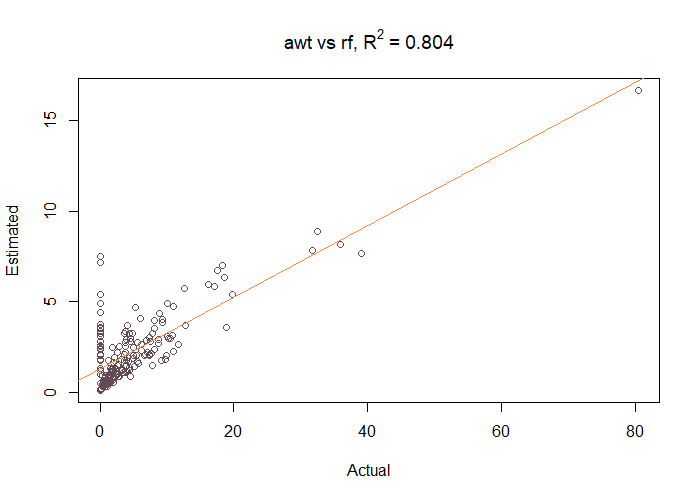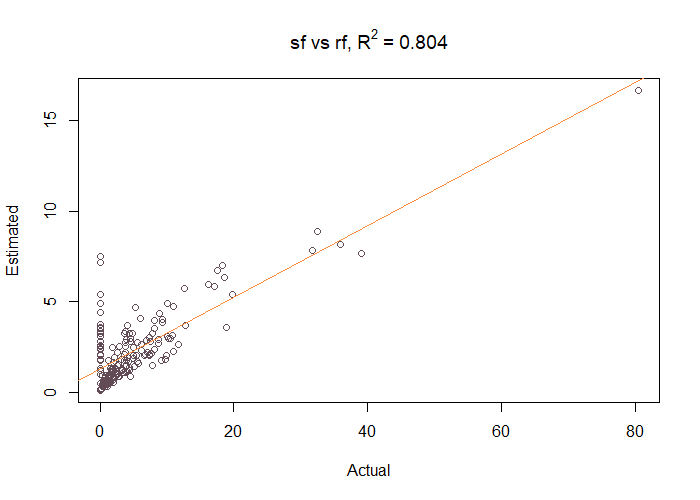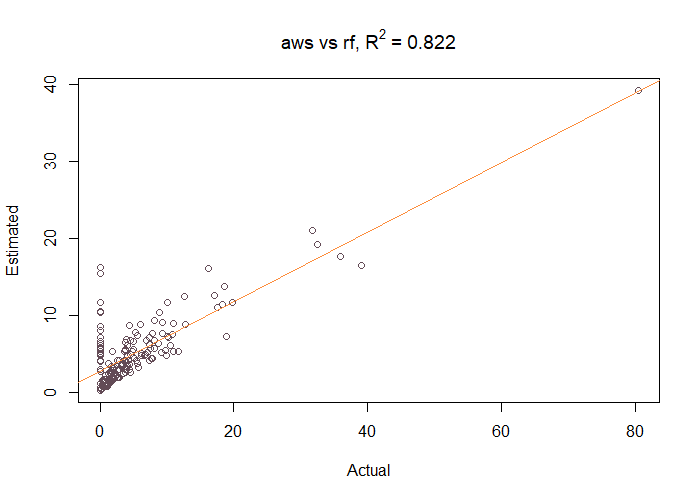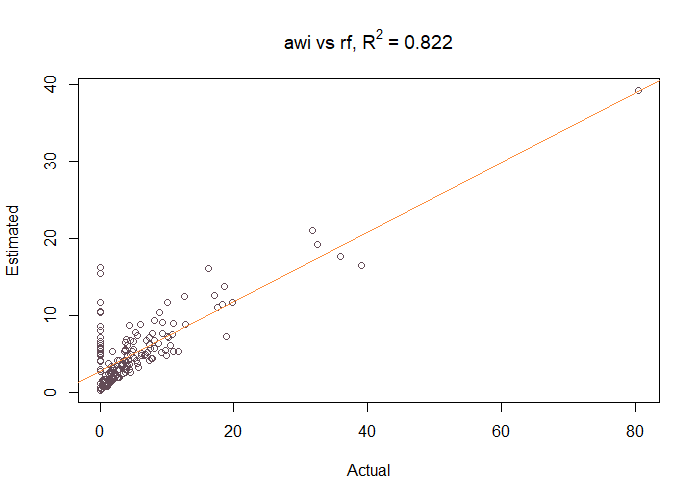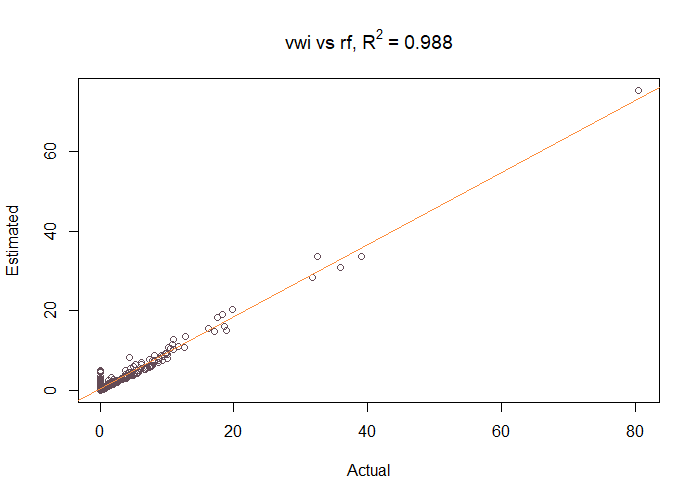Figure 4: Investigation of the relationship of the outcomes and reference data (rf) as the control variable. a) rf vs awt, R2 = 0.804 (top-left), b) rf vs sf, R2 = 0.804 (top-right), c) rf vs aws, R2 = 0.822 (center-left), d) rf vs awi, R2 = 0.822 (center-right) and e) rf vs vwi, R2 = 0.988.

## Performance

In this section, a performance comparison (execution times) takes place using the microbenchmark package. Performance tests are carried out using the sample data provided by populR as well as external data sets from a significantly larger study area (Chios, Greece) with 845 city blocks and 15,946 buildings.

Execution time measurements for both cases are shown in Tables 5 and 6 accordingly. In both cases, execution time measurements suggest that populR performs faster than areal and sf. Using the built-in data, awi and vwi scored the best mean execution time (about 76 milliseconds), which is about 54 millisecond faster than aws, 61 milliseconds faster than sf, and almost 70 milliseconds faster than awt.

Table 5: Execution time measurement comparisons (in milliseconds) using microbenchmark and sample data
Name Min Mean Max
vwi 74.06 76.23 84.39
awi 74.31 75.91 99.70
aws 128.48 132.56 143.97
awt 172.08 176.27 185.86
sf 136.48 142.33 289.38
Table 6: Execution time measurement comparisons (in seconds) using microbenchmark and external data
Name Min Mean Max
vwi 1.55 1.67 1.94
awi 1.55 1.68 1.95
aws 2.26 2.39 2.64
awt 2.27 2.42 2.62
sf 2.63 2.79 3.17

# 4 Conclusions

This study is an attempt to contribute to the continuously growing scientific literature of population downscaling using areal interpolation methods. Despite the fact that there are so many downscaling methods developed, only a few implementation tools are available to the GIS and R community, and therefore, it may be challenging for non-expert GIS users to take advantage of these methods . Due to this lack of implementation tools, this study attempts to fill this gap by introducing populR, an R package for population downscaling using areal interpolation methods.

This package implements two areal interpolation methods, namely awi and vwi. The awi approach guides the interpolation process using the area of intersection between source and target zones while vwi uses the number of floors as additional information to influence the downscaling process. The package is available to the R community though the CRAN and Github repositories.

In order to show the efficacy and efficiency of the introduced package on actual data, a subset area of the city of Mytilini, Greece, was used in the case study. Moreover, a comparative analysis between populR, areal, and sf was carried out, and the results showed that vwi outperformed others by achieving the smallest error measurements, easy implementation and faster execution times. Thus, we strongly believe that R users will gain significant advantage by using populR for population downscaling.

The evaluation of the results indicates the potential of populR in providing better and faster results in comparison to existing R packages. However, we believe that the results may be further improved by incorporating new forms of data, such as Volunteered Geographic Information (VGI), acquired from either social media or open spatial databases such as Open Street Map . By incorporating VGI as ancillary information, we can identify different types of buildings and therefore adjust the weight calculation accordingly. This will be an important improvement that would be helpful for R users interested in population downscaling.

## CRAN Task Views implied by cited packages

T. Ahola, K. Virrantaus, J. M. Krisp and G. J. Hunter. A spatio-temporal population model to support risk assessment and damage analysis for decision-making. International Journal of Geographical Information Science, 21(8): 935–953, 2007. URL https://doi.org/10.1080/13658810701349078.
M. S. Bahadori, A. B. Gonçalves and F. Moura. A systematic review of station location techniques for bicycle-sharing systems planning and operation. ISPRS International Journal of Geo-Information, 10(8): 554, 2021. URL https://doi.org/10.3390/ijgi10080554.
M. Bakillah, S. Liang, A. Mobasheri, J. Jokar Arsanjani and A. Zipf. Fine-resolution population mapping using OpenStreetMap points-of-interest. International Journal of Geographical Information Science, 28(9): 1940–1963, 2014. URL https://doi.org/10.1080/13658816.2014.909045.
M. Batsaris. populR: Population downscaling. 2022. URL https://CRAN.R-project.org/package=populR. R package version 0.1.6.
M. Batsaris, D. Kavroudakis, N. A. Soulakellis and T. Kontos. Location-allocation modeling for emergency evacuation planning in a smart city context. International Journal of Applied Geospatial Research, 10(4): 28–43, 2019. URL https://doi.org/10.4018/ijagr.2019100103.
R. Bian and C. G. Wilmot. Spatiotemporal population distribution method for emergency evacuation. Transportation Research Record: Journal of the Transportation Research Board, 2532(1): 99–106, 2015. URL https://doi.org/10.3141/2532-12.
B. Calka, J. Nowak Da Costa and E. Bielecka. Fine scale population density data and its application in risk assessment. Geomatics, Natural Hazards and Risk, 8(2): 1440–1455, 2017. URL https://doi.org/10.1080/19475705.2017.1345792.
A. Comber and W. Zeng. Spatial interpolation using areal features: A review of methods and opportunities using new forms of data with coded illustrations. Geography Compass, 13(10): 1–23, 2019. URL https://doi.org/10.1111/gec3.12465.
J. E. Dobson, E. A. Bright, R. G. Durfee and B. A. Worley. LandScan: A global population database for estimating population at risk. Photogrammetric Engineering and Remote Sensing, 66(7): 849–857, 2000.
E. European Union. European parliament and council regulation (EC) no 763/2008 on population and housing censuses. 2009. URL https://ec.europa.eu/eurostat/web/population-demography/population-housing-censuses/legislation [online; last accessed September 8, 2021].
F. P. Fisher and M. Langford. Modelling the errors in areal interpolation between zonal systems by monte carlo simulation. Environment and Planning A, 27: 211–224, 1995. URL https://doi.org/10.1068/a270211.
S. Freire and C. Aubrecht. Integrating population dynamics into mapping human exposure to seismic hazard. Natural Hazards and Earth System Science, 12(11): 3533–3543, 2012. URL https://doi.org/10.5194/nhess-12-3533-2012.
M. F. Goodchild and S.-N. N. Lam. Areal interpolation: A variant of the traditional spatial problem. Geo-processing, 1(3): 297–312, 1980.
H. Guo, K. Cao and P. Wang. Population estimation in singapore based on remote sensing and open data. International Archives of the Photogrammetry, Remote Sensing and Spatial Information Sciences - ISPRS Archives, 42: 1181–1187, 2017. URL https://doi.org/10.5194/isprs-archives-XLII-2-W7-1181-2017.
B. Han, M. Hu and J. Wang. Site selection for pre-hospital emergency stations based on the actual spatiotemporal demand: A case study of nanjing city, china. ISPRS International Journal of Geo-Information, 9(10): 542, 2020. URL https://doi.org/10.3390/ijgi9100559.
H. Hellenic Statistical Authority. 2011 population and housing census of greece. Hellenic Statistical Authority. 2014.
H. Kim and X. Yao. Pycnophylactic interpolation revisited: Integration with the dasymetric-mapping method. International Journal of Remote Sensing, 31(21): 5657–5671, 2010. URL https://doi.org/10.1080/01431161.2010.496805.
N. S.-N. Lam. Spatial interpolation methods: A review. The American Cartographer, 10(2): 129–150, 1983. URL https://doi.org/10.1559/152304083783914958.
M. Langford. Rapid facilitation of dasymetric-based population interpolation by means of raster pixel maps. Computers, Environment and Urban Systems, 31(1): 19–32, 2007. URL https://doi.org/10.1016/j.compenvurbsys.2005.07.005.
X. H. Liu, P. C. Kyriakidis and M. F. Goodchild. Population-density estimation using regression and area-to-point residual kriging. International Journal of Geographical Information Science, 22(4): 431–447, 2008. URL https://doi.org/10.1080/13658810701492225.
J. Mennis. Dasymetric mapping for estimating population in small areas. Geography Compass, 3(2): 727–745, 2009. URL https://doi.org/10.1111/j.1749-8198.2009.00220.x.
E. Pebesma. Simple features for R: Standardized support for spatial vector data. R Journal, 10(1): 439–446, 2018. URL https://doi.org/10.32614/rj-2018-009.
C. Prener and C. Revord. areal: An R package for areal weighted interpolation. Journal of Open Source Software, 4(37): 1221, 2019. URL https://doi.org/10.21105/joss.01221.
F. Qiu, C. Zhang and Y. Zhou. The development of an areal interpolation ArcGIS extension and a comparative study. GIScience and Remote Sensing, 49(5): 644–663, 2012. URL https://doi.org/10.2747/1548-1603.49.5.644.
P. Tenerelli, J. F. Gallego and D. Ehrlich. Population density modelling in support of disaster risk assessment. International Journal of Disaster Risk Reduction, 13: 334–341, 2015. URL https://doi.org/10.1016/j.ijdrr.2015.07.015.
W. Wang, Z. Xu, D. Sun and T. Lan. Spatial optimization of mega-city fire stations based on multi-source geospatial data: A case study in beijing. ISPRS International Journal of Geo-Information, 10(5): 282, 2021. URL https://doi.org/10.3390/ijgi10050282.
S.-S. Wu, X. Qiu and L. Wang. Population estimation methods in GIS and remote sensing: A review. GIScience and Remote Sensing, 42(1): 80–96, 2005. URL https://doi.org/10.2747/1548-1603.42.1.80.
X. Yang, T. Ye, N. Zhao, Q. Chen, W. Yue, J. Qi, B. Zeng and P. Jia. Population mapping with multisensor remote sensing images and point-of-interest data. Remote Sensing, 11(5): 574, 2019. URL https://doi.org/10.3390/rs11050574.

### Reuse

Text and figures are licensed under Creative Commons Attribution CC BY 4.0. The figures that have been reused from other sources don't fall under this license and can be recognized by a note in their caption: "Figure from ...".

### Citation

Batsaris & Kavroudakis, "The R Journal: populR: a Package for Population Downscaling in R", The R Journal, 2023

BibTeX citation

@article{RJ-2023-007,
author = {Batsaris, Marios and Kavroudakis, Dimitris},
title = {The R Journal: populR: a Package for Population Downscaling in R},
journal = {The R Journal},
year = {2023},
note = {https://doi.org/10.32614/RJ-2023-007},
doi = {10.32614/RJ-2023-007},
volume = {14},
issue = {4},
issn = {2073-4859},
pages = {223-234}
}